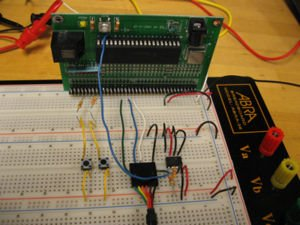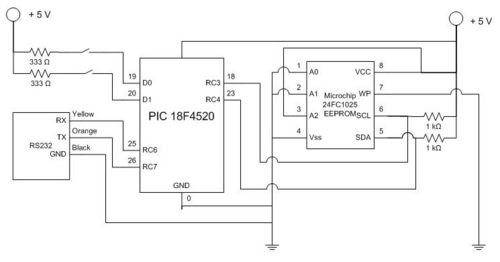# Data logging with an EEPROM

##Original Assignment

Your task is to use the PIC to log data from an analog input on an EEPROM, and after the data collection is over, to send the data back from the EEPROM to a PC running matlab. Use an interrupt service routine to read an analog input and write the value to an external EEPROM using I2C. After reading in a fixed number of samples, perhaps 1,000 or 10,000, the program should send the data back to a matlab program via an RS-232 link. The logged data should then be plotted by matlab.

You should decrease the sample time until the ISR does not successfully complete. What is the fastest rate at which an analog input can be read and stored to the external EEPROM? Try this for an analog input configured for both 8-bit (1 byte of data stored on the EEPROM) and 10-bit (2 bytes of data stored on the EEPROM). How much data can you store on the EEPROM?

As an alternative, you can avoid the use of an ISR and simply read and store the data as fast as you can in a loop.

As a test input, use a triangle wave signal from the function generator between 1 and 4 V, and plot the data in matlab.

## Overview

An external EEPROM is useful when trying to store data. In addition to storing much more data than is available on the 18F4520 PIC, an EEPROM stores the data even when power is removed and can then be collected at a later time. Storing large amounts of data over time is especially beneficial once communication can be made between the PIC and Matlab. Using the serial function in Matlab, the data can be obtained and then analyzed.
In this project, we used the PIC to log data from an analog input onto an EEPROM and then later sent the data back from the EEPROM to Matlab to plot. For our lab, we worked with a 24FC1025 EEPROM whose data sheet can be found here. Additionally, we established serial port connection between the PIC microcontroller and Matlab using the RS232. Our project also made use of two buttons. The first button tells the PIC to begin collecting data points from the analog input and storing the data onto the EEPROM. The second button tells the PIC to send the data from the EEPROM to Matlab.

##Circuit

To the right is a circuit diagram for interfacing a 24FC1025 EEPROM to the 18F4520 PIC. Below the diagram are photos of the circuit layout.
inputs on the PIC which dictate when to collect and store data and when to send the data to Matlab.

For more detail: Data logging with an EEPROM